• 216
•
•
•
•
•
•
•
216
Shares

# MASS ENERGY EQUIVALENCE

Today we are going to talk about a famous formula, which has changed the thinking of the world and who gives the underlying concept of time travel. We are talking about the mass-energy equivalence ie. E = mc^2.

This formula is given by Albert Einstein on 21 November 1905, in the paper “Does the inertia of a body depends upon its energy content”  mass-energy equivalence is a product of the special theory of relativity, and physicist Henry Poincaré described it as a paradox.Mass energy equivalence is a very important principle of relativistic physics, which gives a relation between mass and energy.

This principle tells us that anything which have mass has an equivalent amount of energy and vice versa, vice versa means if a mass contains energy then energy contains mass. And these fundamental quantities are related to each other by Einstein’s famous formula ie. E = mc^2. Where c is the speed of light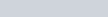.

According to this formula, if anyone wants to calculate the energy of a body having mass m then he has to multiply it with the squared of the speed of light ie. c^2. It means if anyone is traveling with the squared of the speed of light then, then he has been converted into energy.

Then from this, we can say that mass can’t exist without energy, and energy can’t exist without mass. If energy exists then somewhere mass will also exist, and if the mass is present then energy will also present because we know that energy can’t exist without mass.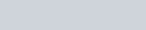The energy content of anybody is directly proportional to its mass, if the mass is large then energy corresponding to it is also large.

And we have seen in the special theory of relativity that mass is a relativistic quantity, the mass of the body increases if its velocity is increasing. And here conversation of mass into energy is occurring at the speed of light, which means the mass of the body is approximately infinite. Everybody has its two mass, one when it is in rest and one when it is in motion.When it is in rest, then mass corresponding to it is called rest mass or invariant mass.

This mass is invariant to all frames of reference. But when the body is in motion then its mass changes and the variation of mass due to motion (relativity of mass) and mass corresponding to this motion is called relativistic mass. Which is given as-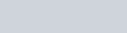When a body is in motion then its total energy is greater than its rest energy.
Now we are going to derive the formula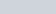.

## DERIVATION FOR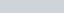Consider the relationSquaring both sides we get-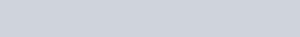we get-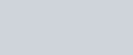Now differentiate it, then we get-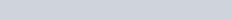Then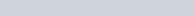Cancelling 2m from both side, we get-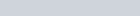Now take a small kinetic energy dk for small velocity, Then-Putting the value of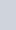into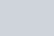, we get-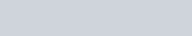And can also written as-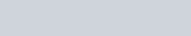Then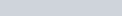And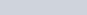it is equal to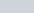Then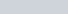So now integrate it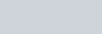We get-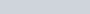And we can write PurleyThis is the derivation for the mass-energy equivalence i.e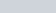.